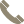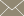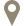Home » Faculty Profiles » Faculty

### Wang, Hongwei

Assistant Professor

#### CONTACT INFO(956) 326-2413hongwei.wang@tamiu.eduLBV-315

#### COURSES TAUGHT

• Applied Data Analysis (STAT 5305)
• Business Math II (MATH 1325)
• Calculus II (MATH 2414)
• Calculus III (MATH 2415)
• Categorical Data Analysis (STAT 5300)
• College Algebra (MATH 1314)
• Differential Geometry (MATH 5340)
• Engineering Statistics (ENGR 2372)
• Generalized Lin Mdls with Apps (STAT 5306)
• Graph Theory (MATH 5316)
• Introduction to Topology (MATH 4360)
• Introductory Statistics (MATH 1342)
• Plane Trigonometry (MATH 1316)
• Pre-Calculus (MATH 2412)
• Quality Control & Improvement (STAT 5340)
• Stat Methods for Clin Trials (STAT 5310)
• Statistical Analysis (MATH 3360)
• Topology (MATH 5365)